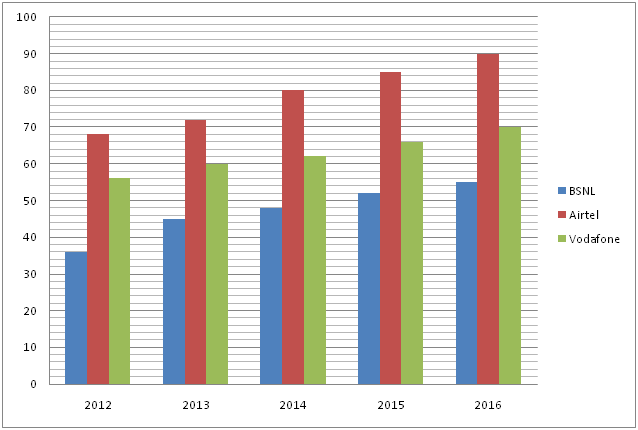# CWC/FCI Prelims 2019 – Quantitative Aptitude Questions (Day-21)

Dear Readers, Exam Race for the Year 2019 has already started, To enrich your preparation here we are providing new series of Practice Questions on Quantitative Aptitude – Section for CWC/FCI Exam. Aspirants, practice these questions on a regular basis to improve your score in aptitude section. Start your effective preparation from the right beginning to get success in upcoming CWC/FCI Exam.

[WpProQuiz 5633]

Directions (Q. 1 – 5): What value should come in place of (?) in the following questions?

1) (2370 ÷ 15) × 10 + 33 % of 1800 – (4/7) of 2170 =? + 44

a) 560

b) 670

c) 890

d) 720

e) None of these

2) ? % of 500 – 8 % of 3000 – (3/8) of (2/3) of 72 = 23

a) 56.2

b) 45.8

c) 51.4

d) 42.6

e) None of these

3) 242 ÷ 42 × 120 ÷ 10 =? – 552 ÷ 4

a) 620

b) 645

c) 535

d) 570

e) None of these

4) 97336 + 48 % of 1050 – (2/3) of 726 = ?

a) 75

b) 66

c) 52

d) 84

e) None of these

5) 11 (5/8) + 4 (7/16) – 9 (3/8) + 12 (3/16) = ? – 3 (7/8)

a) 22 (3/4)

b) 19 (5/8)

c) 21 (¼)

d) 18 (½)

e) None of these

Directions (Q. 6 – 10): Study the following information carefully and answer the given questions:

The following bar graph shows the total number of peoples (In lakhs) using three different types of mobile services over the years in a certain state.6) Find the average number of people using Airtel services all the given years together?

a) 83 lakhs

b) 66 lakhs

c) 79 lakhs

d) 72 lakhs

e) None of these

7) Total number of people using all three given services together in the year 2012 is approximately what percentage of total number of people using all three given services together in the year 2014?

a) 100 %

b) 84 %

c) 70 %

d) 115 %

e) 130 %

8) Find the difference between the total number of people using BSNL services in all the given years together to that of total number of people using all three given services together in the year 2013 and 2015 together?

a) 123 lakhs

b) 136 lakhs

c) 117 lakhs

d) 144 lakhs

e) None of these

9) Find the ratio between the total number of people using Vodafone in the year 2012, 2014 and 2016 together to that of total number of people using all three given services together in the year 2016?

a) 188: 215

b) 124: 173

c) 138: 197

d) 225: 289

e) None of these

10) Total number of people using Vodafone in the year 2013 and 2016 together is what percentage more than total number of people using Airtel in the year 2014?

a) 62.5 % less

b) 62.25 % less

c) 62.5 % more

d) 62.25 % more

e) None of these

Direction (1-5) :

(2370/15)*10 + (33/100)*1800 – (4/7)*2170 = x + 44

1580 + 594 – 1240 – 44 = x

X = 890

(x/100)*500 – (8/100)*3000 – (3/8)*(2/3)*72 = 23

5x – 240 – 18 = 23

5x = 23 + 240 + 18

5x = 281

X = 281/5 = 56.2

242 ÷ 42 × 120 ÷ 10 = x – 552 ÷ 4

[(24*24)/(4*4)]*(120/10) = x – (552/4)

432 + 138 = x

X = 570

∛97336 + 48 % of 1050 – (2/3) of 726 = x

X = 46 + (48/100)*1050 – (2/3)*726

X = 46 + 504 – 484 = 66

11 (5/8) + 4 (7/16) – 9 (3/8) + 12 (3/16) =? – 3 (7/8)

X = (11 + 4 – 9 + 12 + 3) (5/8 + 7/16 – 3/8 + 3/16 + 7/8)

X = 21 (28/16) = 21(7/4) = 22 (3/4)

Direction (6-10) :

The total number of people using Airtel services all the given years together

= > 68 + 72 + 80 + 85 + 90

= > 395 lakhs

Required average = 395/5 = 79 lakhs

Total number of people using all three given services together in the year 2012

= > 36 + 68 + 56 = 160 lakhs

Total number of people using all three given services together in the year 2014

= > 48 + 80 + 62 = 190 lakhs

Required % = (160/190)*100 = 84.21 % = 84 %

The total number of people using BSNL services in all the given years together

= > 36 + 45 + 48 + 52 + 55 = 236 lakhs

The total number of people using all three given services together in the year 2013 and 2015 together

= > 45 + 72 + 60 + 52 + 85 + 66 = 380 lakhs

Required difference = 380 – 236 = 144 lakhs

The total number of people using Vodafone in the year 2012, 2014 and 2016 together

= > 56 + 62 + 70 = 188

The total number of people using all three given services together in the year 2016

= > 55 + 90 + 70 = 215

Required ratio = 188: 215

Total number of people using Vodafone in the year 2013 and 2016 together

= > 60 + 70 = 130 lakhs

Total number of people using Airtel in the year 2014 = 80 lakhs

Required % = [(130 – 80)/80]*100 = 62.5 % more